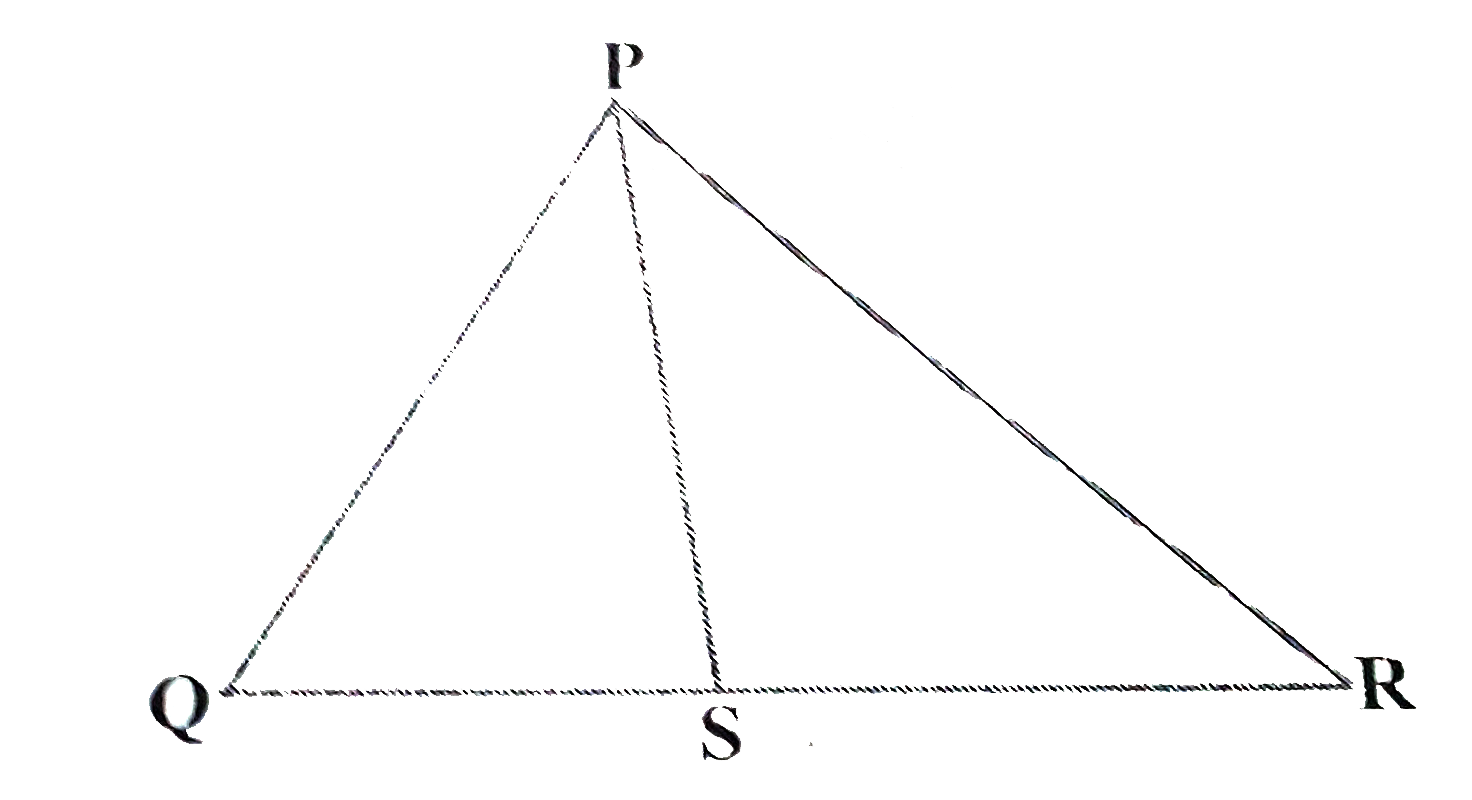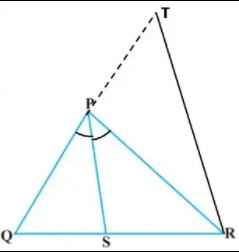Question

Updated on:26/04/2023

# In figure PS is the bisector of ∠QPRof ΔPQR. Prove that QSSR=PQPR.Text Solution

Solution
In QRT RTSP [By construction] And PS intersects QT and QR at two distinct points P and Q.
Therefore,
QT and QR will be divided in the same ratio
QSSR=PTPQ......(1)
[Basic proportionality theorem: If a line is drawn parallel to one side of a triangle, intersecting the other two sides at distinct points, then the other two sides are divided in the same ratio.]
Now, RTSP
and PR is the transversal
Therefore,
SPR=PRT....(2)
[Alternate interior angles] and QPS=PTR....(3)
[Corresponding angles]
Also, given the PS is the bisector of QPRQPR=SPR
From (2) and (3)
PTR=PRT
Therefore, PT=PR [Sides opposite to equal angles of a triangle are equal]
Putting PT=PR in (1)
QSSR=PQPT
Hence proved.